Get instant live expert help with Excel or Google Sheets“My Excelchat expert helped me in less than 20 minutes, saving me what would have been 5 hours of work!”

#### Post your problem and you’ll get expert help in seconds.

Your message must be at least 40 characters
Our professional experts are available now. Your privacy is guaranteed.

# Learn How to Use the TAN Function in ExcelRead time: 20 minutes

We can find the TANGENT of an angle by using the TAN function and the RADIANS function. This can also be done by using the TAN and PI function.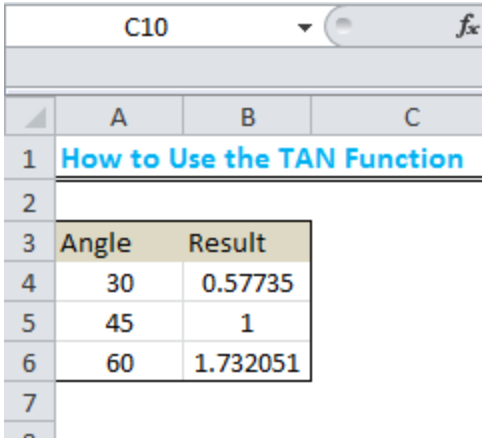Figure 1: Result of the TAN function for 30, 45, and 60 degrees

## Using the TAN and RADIANS function

The TAN function returns the tangent of an angle that is in RADIANS. Hence, for us to use the TAN function here, we must first convert the angle to RADIANS.

## Syntax

`=TAN(RADIANS(ANGLE))`

## Setting up the Data

We will find the tangent of the following angles by using the TAN function; 30, 45 and 60 degrees.

• We will place the angles in Column A
• Our formula will be placed in Column B to display the result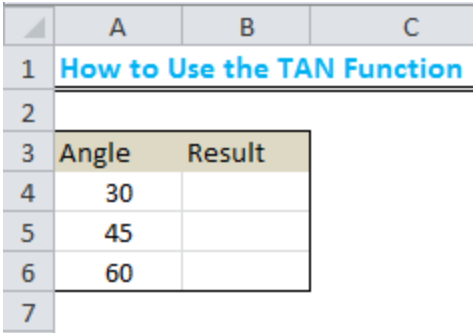Figure 2: How to use the TAN function

## The Result

• We will input the formula below into Cell B4:

`=TAN(RADIANS(A4))`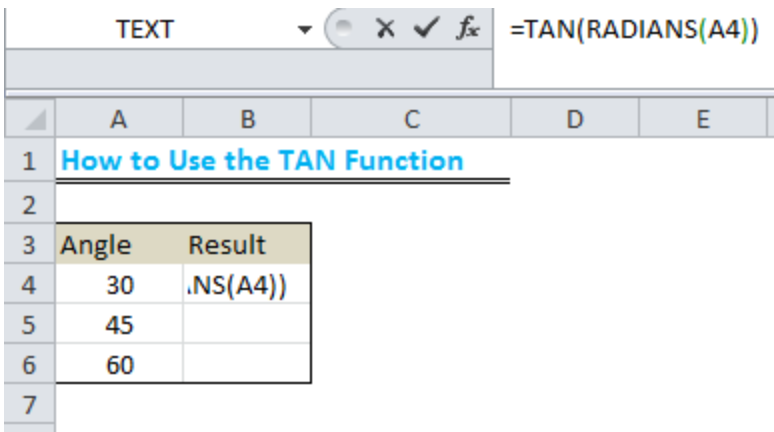Figure 3: How to use the TAN function

• We will now press ENTER to obtain the result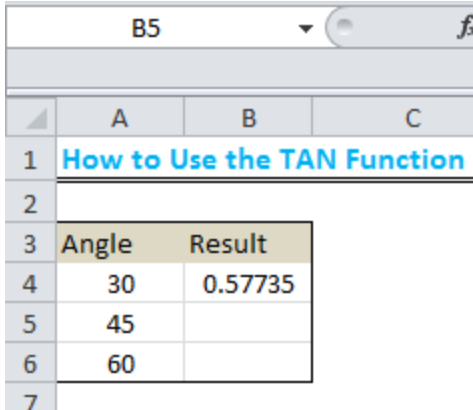Figure 4: Result of the TAN function using Radians

• We will use the drop-down feature to get the results for 45 and 60 degrees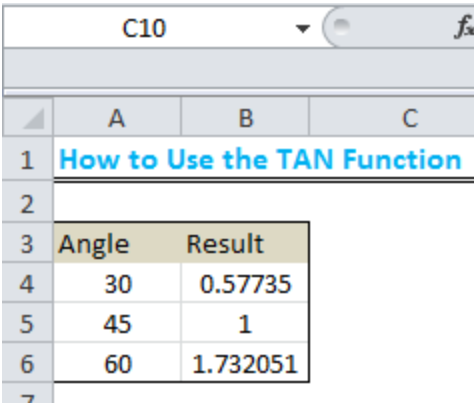Figure 5: Result of the TAN function for 30, 45, and 60 degrees

## Using the PI function

We can also use the PI function to tackle this. The formula with the PI function is given below:

`=TAN(A4*PI()/180)`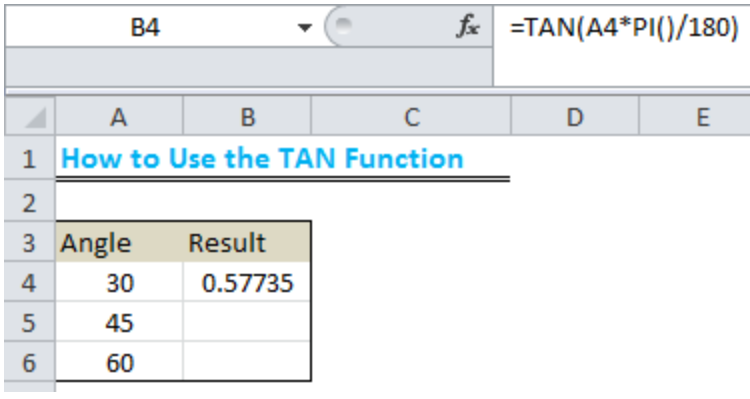Figure 6: Using the PI function for 30 degrees

As done before, we will use the drop-down arrow to get the result for 45 and 60 degrees.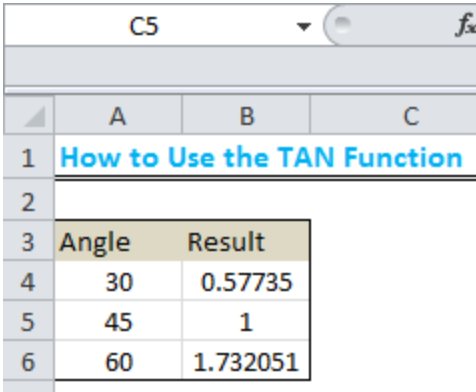Figure 7: Result of the TAN function with the PI function for 30, 45, and 60 degrees

## Instant Connection to an Expert through our Excelchat Service

Most of the time, the problem you will need to solve will be more complex than a simple application of a formula or function. If you want to save hours of research and frustration, try our live Excelchat service! Our Excel Experts are available 24/7 to answer any Excel question you may have. We guarantee a connection within 30 seconds and a customized solution within 20 minutes.

### Did this post not answer your question? Get a solution from connecting with the expert.Another blog reader asked this question today on Excelchat:
Related blogs
Solution examplesColumn A "Date Received" Column C "Priority" is validation list with options P1-P6 Column D "Due Date" .. How do I make Column D calculate the due date, based on which option is selected in Column C in conjunction with the date in Column A? PI = Date Received P2 = Date Received P3 = Date Received + 1 day P4 = Date Received + 5 business days P5 = Date Received + 14 business days P6 = Date Received + 30 business days
Solved by D. U. in 15 minsI am working in excel version 15.41 I want to compare to two large lists of doctors to determine matches based on NPI numbers. List are on 2 separate sheets (sheet 1 Column G and Sheet 2 Column A). I would like the sheet with duplications to be shown on Sheet 1 by highlighting the matching NPI numbers and lines that match. However I can not load the list because the file exceeds 20MB
Solved by E. L. in 60 minsHow can I create PIVOT Table for a large data having merged cell and free spaces, please
Solved by Z. L. in 29 minsIf I merged a multi-row, multi-column selection. Iand types some text into the merged cell and then unmerges it, what will happen to the text?
Solved by S. Y. in 60 mins## Subscribe to Excelchat.coAnother blog reader asked this question today on Excelchat: